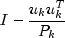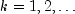JMSLTM Numerical Library 7.2.0
com.imsl.math

## Class QR

• All Implemented Interfaces:
Serializable, Cloneable

```public class QR
extends Object
implements Serializable, Cloneable```
QR Decomposition of a matrix.

Class `QR` computes the QR decomposition of a matrix using Householder transformations. It is based on the LINPACK routine `SQRDC`; see Dongarra et al. (1979).

`QR` determines an orthogonal matrix Q, a permutation matrix P, and an upper trapezoidal matrix R with diagonal elements of nonincreasing magnitude, such that AP = QR. The Householder transformation for column k is of the formfor, min(number of rows of A, number of columns of A), where u has zeros in the first k - 1 positions. The matrix Q is not produced directly by `QR`. Instead the information needed to reconstruct the Householder transformations is saved. If the matrix Q is needed explicitly, the method `getQ` can be called after `QR`. This method accumulates Q from its factored form.

Before the decomposition is computed, initial columns are moved to the beginning of the array A and the final columns to the end. Both initial and final columns are frozen in place during the computation. Only free columns are pivoted. Pivoting is done on the free columns of largest reduced norm.

Example, Serialized Form
• ### Constructor Summary

Constructors
Constructor and Description
`QR(double[][] a)`
Constructs the QR decomposition of a matrix with elements of type `double`.
• ### Method Summary

Methods
Modifier and Type Method and Description
`int[]` `getPermute()`
Returns an integer vector containing information about the permutation of the elements of the matrix during pivoting.
`double[][]` `getQ()`
Returns the orthogonal or unitary matrix Q.
`double[][]` `getR()`
Returns the upper trapezoidal matrix R.
`int` `getRank()`
Returns the rank of the matrix used to construct this instance.
`int` `rank(double tolerance)`
Returns the rank of the matrix given an input tolerance.
`double[]` `solve(double[] b)`
Returns the solution to the least-squares problem Ax = b.
`double[]` ```solve(double[] b, double tol)```
Returns the solution to the least-squares problem Ax = b using an input tolerance.
• ### Methods inherited from class java.lang.Object

`clone, equals, finalize, getClass, hashCode, notify, notifyAll, toString, wait, wait, wait`
• ### Constructor Detail

• #### QR

`public QR(double[][] a)`
Constructs the QR decomposition of a matrix with elements of type `double`.
Parameters:
`a` - a `double` matrix to be factored
Throws:
`IllegalArgumentException` - Thrown when the row lengths of input matrix a are not equal (i.e. the matrix edges are "jagged".)
• ### Method Detail

• #### getPermute

`public int[] getPermute()`
Returns an integer vector containing information about the permutation of the elements of the matrix during pivoting.
Returns:
an `int` array containing the permutation information. The k-th element contains the index of the column of the matrix that has been interchanged into the k-th column.
• #### getQ

`public double[][] getQ()`
Returns the orthogonal or unitary matrix Q.
Returns:
a `double` matrix containing the accumulated orthogonal matrix Q from the QR decomposition
• #### getR

`public double[][] getR()`
Returns the upper trapezoidal matrix R.
Returns:
the upper trapezoidal `double` matrix R of the QR decomposition
• #### getRank

`public int getRank()`
Returns the rank of the matrix used to construct this instance.
Returns:
an `int` specifying the rank of the matrix used to construct this instance
• #### rank

`public int rank(double tolerance)`
Returns the rank of the matrix given an input tolerance.
Parameters:
`tolerance` - a `double` scalar value used in determining the rank of the matrix
Returns:
an `int` specifying the rank of the matrix
• #### solve

```public double[] solve(double[] b)
throws SingularMatrixException```
Returns the solution to the least-squares problem Ax = b.
Parameters:
`b` - a `double` array to be manipulated
Returns:
a `double` array containing the solution vector to Ax = b with components corresponding to the unused columns set to zero
Throws:
`SingularMatrixException` - Thrown when the upper triangular matrix R resulting from the QR factorization is singular.
• #### solve

```public double[] solve(double[] b,
double tol)
throws SingularMatrixException```
Returns the solution to the least-squares problem Ax = b using an input tolerance.
Parameters:
`b` - a `double` array to be manipulated
`tol` - a `double` scalar value used in determining the rank of A
Returns:
a `double` array containing the solution vector to Ax = b with components corresponding to the unused columns set to zero
Throws:
`SingularMatrixException` - Thrown when the upper triangular matrix R resulting from the QR factorization is singular.
JMSLTM Numerical Library 7.2.0